×#### Thank you for registering.

One of our academic counsellors will contact you within 1 working day.

Click to Chat

1800-1023-196

+91-120-4616500

CART 0

• 0

MY CART (5)

Use Coupon: CART20 and get 20% off on all online Study Material

ITEM
DETAILS
MRP
DISCOUNT
FINAL PRICE
Total Price: Rs.

There are no items in this cart.
Continue Shopping• Complete Physics Course - Class 11
• OFFERED PRICE: Rs. 2,968
• View Details

```Introduction of Electrostatic Potential and Capacitance

Table of Content

What is Electrostatic?

Electrostatic Charge

Electrostatic Potential

Coulomb’s Law

Laws of governing Electrostatics

Potential Gradient and Electric Field Intensity

Equipotential Surfaces

Electric Potential Energy

Electrostatic Shielding

What is Capacitor?

Capacitance in Physics

Capacitor in Series and in Parallel

Electrical Capacitance

Parallel Plate Capacitor

Capacity of Parallel Plate Capacitor

Effect of Dielectric on Capacitance of Capacitor

Energy Stored on a Capacitor

Energy Density

Numericals

What is Electrostatic?

Electrostatic is a branch of physics which deals with characteristics electric charges which are slow moving or stationary.

Examples of Electrostatic Phenomenon are:

Laser machine and photocopier

Spontaneous explosion of grain silos

Attraction of paper to a charger scale

In our childhood, we rubbed the scale on our head and try to attract paper from that scale. This is an example of Electrostatic Phenomenon.

Electrostatic Charge

We are familiar with charge flowing through conductors, which we usually just call "electricity". Charge can also be present on insulators and because
these materials do not allow the charge to flow, this
is called Electrostatic Charge

Charges are of two types:

Positive Charge

Negative Charge

This depends upon the addition or removal of electrons from an atom. If there are more protons than electrons the atom is positively charge and if there are more electrons than protons than the atom is negatively charge.Fig 1.1 Line of forces for positively and negatively charged particles

Line of Force: A theoretical line in a field of force, such that a tangent at any point gives the direction of the field at that point.

Electrostatic Potential

Inside a field, the amount of work need to move a unit positive charge from one initial point to any specified point without producing any acceleration is known as Electrostatic Potential (or also known as Electric Potential and Electric Field Potential).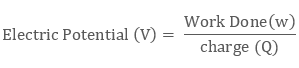Usually the initial or starting point is Earth or a point which is at infinity.

Few points to keep in mind about electric potential are:

Electrostatic potential is denoted by V and it is a scalar quantity.

Electrostatic potential can be measured in volts.

Coulomb’s Law

The coulomb’s law of electrostatics is also known as Coulomb’s Inverse Square Law which describes the forces acting between the static electrically charged particles.

In its scalar form the law can be written as: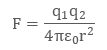Laws of governing Electrostatics

First Law: The first law states of electrostatic state that ,like charges repel each other while unlike charges attract each other that is two positive charges will repel each other while one positive and one negative charge will attract each other.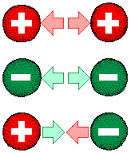Fig 1.2 Like and Unlike Charges

Second Law: The second law states the properties exerted between two point charges: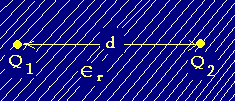Fig 1.3 shows two point charge separated by distance d

is directly proportional to the product of their strengths.

is inversely proportional to the square of the distance between them.

is inversely proportional to the absolute permittivity of the surrounding medium.

This is known as Coulomb's Law.

Potential Gradient and Electric Field Intensity

The rate at which electrostatic charge is changed with respect to displacement is known as Potential Gradient.

Electric field intensity is the negative of potential gradient at that point.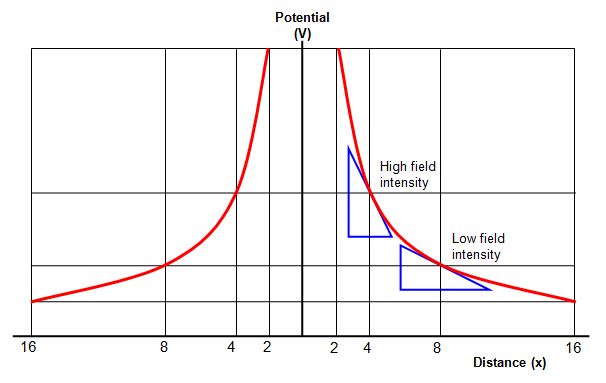Fig 1.5 Potential gradient and electric field intensity for a radial field

Equipotential Surfaces

The surfaces which have equal electrostatic potential at each point are known as Equipotential Surfaces.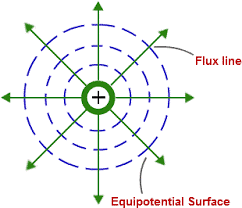Fig 1.4 Equipotential Surface

The work done to move any charge from one place to other place on an equipotential surface is zero as all the points have same potential.

Electric Potential Energy

The potential energy that results from conservative Coulomb forces and is associated with the configuration of a particular set of point charges within a defined system is known as Electric Potential Energy and is measured in joules.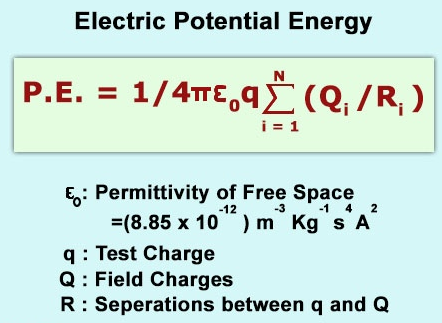Fig 1.6 Electrostatic potential Energy

Electrostatic Shielding

Electrostatic Shielding is the process of setting apart a certain region of space from external field. It is based on the fact that the electric field in conductor is zero.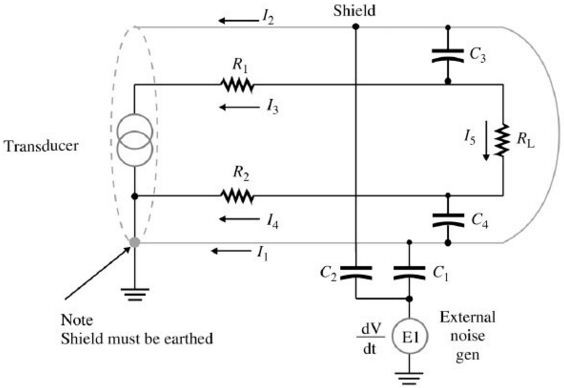Fig 1.7 Electrostatic Shielding

What is Capacitor?

Capacitor also known as Condenser is two terminal electric components which has ability or capacity to store energy in the form of electric charge. The storing capacity of capacitance very from small storage to high storage.Fig 1.8 Capacitors

Capacitance in Physics

The ability of ancapacitor to store the energy in form of electric charge is known as Capacitance.

In other words, capacitance can be described as the storing ability of an capacitor and it is measured in farads.

For Example, if we connected a capacitor to a 9 volt battery and measured that it stored 9 coulombs of charge, its capacitance would be 1 farad.

Capacitor in Series and in Parallel

In Series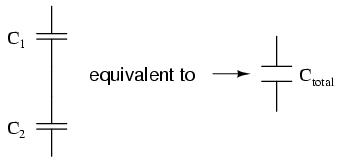Fig 1.9 capacitors connected in series

Capacitor C1 and C2 are connected in series. When the capacitors are connected serially than the total capacitance that is Ctotal is less any one of the capacitor’s capacitance.

The capacitance of capacitor connected in series can be calculated as: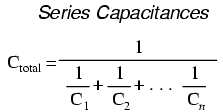In ParallelFig 1.10 Capacitor connected in parallel

Capacitor C1 and C2 are connected in parallel. When the capacitors are connected parallel than the total capacitance that is Ctotalis any one of the capacitor’s capacitance

The capacitance of capacitor connected in parallel can be calculated as:Electrical Capacitance

Electrical conductance of a conductor is defined as the capacity to store charge in it. Whenever charge is applied to an insulator its potential is raised to some certain level. Charge on a conductor and its electric potential are both directly proportional to each other. So, as we increase the charge electric potential also increases.

V ∝ Q
Or
Q = C V

C is the proportionality constant

Parallel Plate Capacitor

The setup of dielectric and electrode to form a capacitor is known as parallel plate capacitor.

The two parallel conductive plates (electrodes) are used and in between medium of dielectric. The value of capacitance is dependent on the material or the medium between the two platesFig 1.11 Parallel Plate Capacitor

A is the common area of the two plates and d is the distance between the plates.

Capacity of Parallel Plate Capacitor

Cair = ε0A/d

Cmed = Kε0A/d

Here, A is the common area of the two plates and d is the distance between the plates.

Effect of Dielectric on Capacitance of Capacitor

Faraday have given this theory, that is if the space between two electrodes are filled with dielectric the capacitance of the capacitor will be increased.

If all the space is filled by a dielectric that is there is no place left then the capacitance of a capacitor will be increased by K. and here K is known as Dielectric Constant.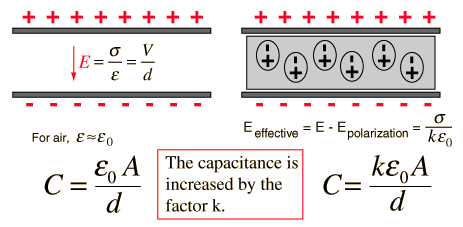Fig 1.12 Effect of Dielectric on Capacitor

Energy Stored on a Capacitor

Energy Stored on a Capacitor can be calculated through following expression: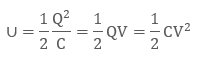Energy Density

The total amount of energy per unit volume is known as Energy Density.

Energy density is denoted by U.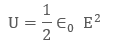Numericals

(Related to coulomb’s law, electrostatic potential)

1. A point charge q = - 8.0 × 10-9 C is placed at the origin. Calculate electric field at a point 2.0 m from the origin on the z-axis.

Given data: Charge = q = -8.0 × 10-8 C

Distance = r = 2.0m

To be found: E =?

Calculations: We know that, by coulomb’s lawPutting values, we get:

E= 180N C-1

Since point is on z-axis

E = 180 NC-1 ќ

2. A particle having a charge of 20 electrons on it falls through a potential difference of 100 volts. Calculate the energy acquired by it in electron volts (eV)

Given Data: Charge = q = 20

e = 20 1.6 10-9 = 32 × 10-19C

Potential difference = ΔV = 100 V

To determine: Energy required in (eV) = E =?

Calculations:

We know that:

E = qΔV

= (32× 10-19)(100)

= 3200 × 10-19 Joules

Since 1eV = 1.6 × 10-19C

= 2000eV

E = 2 × 103 eV

(Related to energy density and parallel plate capacitors)

1. Find the energy density of a capacitor if its electric field, E = 5 V/m.

Given,

E = 5V/m

We know that,

ϵ0 = 8.8541 × 10−12 F/m

The energy density formula of capacitor is given by

U = 1 / 2 ϵ0E2

= 1 / 2 × 8.8541 × 10−12 × 52

U = 1.10×10−10 FV2/m3

2. A parallel plate capacitor is placed in the air has an area of 0.44m22 and are separated from each other by distance 0.04m. Determine the parallel plate capacitor

Given: Area A = 0.44 m22,

distance d = 0.04 m,

relative permittivity k = 1,

ϵo= 8.54 ×10−12 F/m

The parallel plate capacitor formula is given by,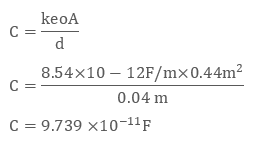Therefore, the parallel plate capacitor is9.739 × 10-11 F

Watch this Video for more reference

Introduction of Electrostatic Potential and Capacitanc
```### Course Features

• 101 Video Lectures
• Revision Notes
• Previous Year Papers
• Mind Map
• Study Planner
• NCERT Solutions
• Discussion Forum
• Test paper with Video Solution